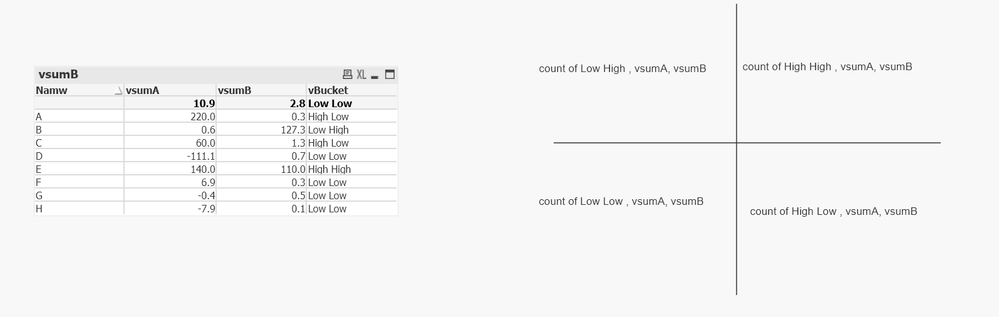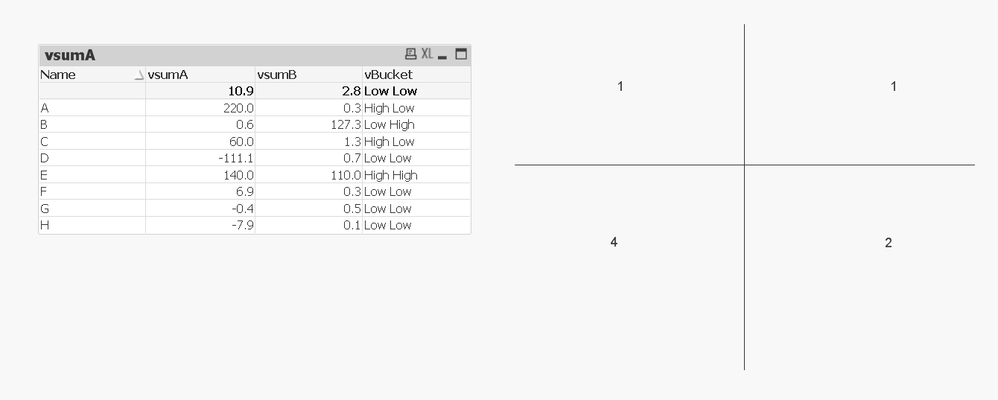# QlikView App Development

Discussion Board for collaboration related to QlikView App Development.

Announcements
Modernize Your QlikView Deployment webinar, Nov. 3rd. REGISTER
cancel
Showing results for
Did you mean:
HighlightedCreator

## Varaibles in Set analysis

Hi,

I have below expressions:

vBucket = if((\$(vsumA) < \$(vA_hurdle) or trim(len(\$(vsumA))) <=1) and (\$(vsumB) < \$(vB_hurdle) or trim(len(\$(vsumB)))<=1),  'Low Low',
if((\$(vsumA) < \$(vA_hurdle) or trim(len(\$(vsumA))) <=1) and \$(vsumB) > \$(vB_hurdle),         'Low High',
if(\$(vsumA) > \$(vA_hurdle)         and (\$(vsumB) < \$(vB_hurdle) or trim(len(\$(vsumB)))<=1),  'High Low',
if(\$(vsumA) > \$(vA_hurdle)         and \$(vsumB) > \$(vB_hurdle),         'High High'))))

vsumA = sum(A_num)/sum(A_den)

vsumB = sum(B_num)/sum(B_den)I want to show following summary: count, vSumA and vSumB for each bucket

This summary shouldn't change based on user selection.

I tried multiple things but couldn't find any solution.

Kindly help!

Thanks

Labels (2)

• ### Summary Set Expression in Text Object

1 Solution

Accepted Solutions
HighlightedMVP

This?2 Replies
HighlightedMVP

This?HighlightedCreator

Thanks Sunny 🙂